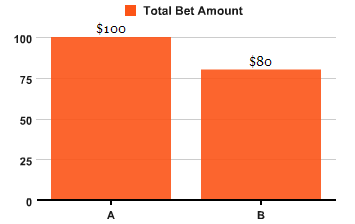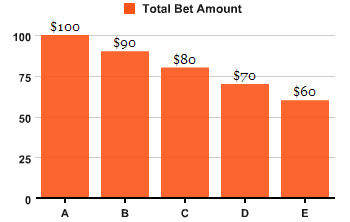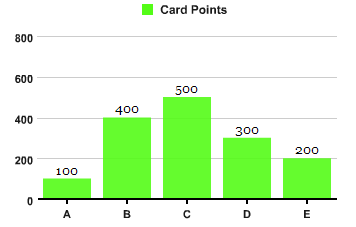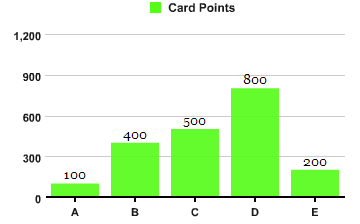# You good at math? Help me figure out a formula/solution!

Before going into PHP & MySQL, let me story a bit. This is a problem of Texas Holdem game project I am working on, and of course you don’t need a math major.

We have 2 players now at the moment, Player A and player B.

A has total bet of \$100
B has total bet of \$80According to Texas Holdem betting rules, whenever there is a different in total bet amount, a side pot is created.

So, our example above will creat a side-pot of \$20, with main-pot of \$80 + \$80 = \$160

Main-Pot = \$160, contributed by both A & B
Side-Pot = \$20, contributed by A only

If Player B win the hand, he will take back \$160(the main-pot), Player A will take back \$20 since he is the only one has the right of the side-pot.

This is easy.

Now let’s look at more players, say 5 players, each with total bet amount of the following:–

Player A = \$100
Player B = \$90
Player C = \$80
Player D = \$70
Player E = \$60Let’s calculate main-pot, main-pot is always the smallest bet amount times number of players, that is \$60 * 5 = \$300

Side-pots? OMG! This is headache, really!

player A bet amount \$100, B \$90, a different of \$10. Thus we have Side-Pot_1 pot size of \$10, only A has right to it.

Comes Player C of \$80. Look at the graph above, diff between C(\$80) and B(\$90) is \$10, different between B(\$90) and A(\$100) is \$10, thus we have Side-Pot_2 pot size of \$10 + \$10 = \$20, A and B have right to it.

Just imagine a horizontal dotted line at top of C bar and cut through B and A bars. If C wins, he can only win max. of \$80 from B as well as \$80 from A, and all of \$70 from D and \$60 from E.

Leaving a surplus of \$30, where \$10 are claimed by A only, and \$20 which A & B have the right to it.

Then come Player D, Player E. Side-pots are created like above mentioned

Main-Pot = \$300, every players have the right.

Side-Pot_1 = \$10, Player A has the right.
Side-Pot_2 = \$20, contributors A & B.
Side-Pot_3 = \$30, contributors A & B & C.
Side-Pot_4 = \$40, contributors A & B & C & D.

The winner is found with highest card-points. Now let’s say Player C has highest card points follow by B, then D, then E, then ALet’s calculate total Player C can take back.

Main-Pot of \$300
Side-Pot_3 of \$30
Side-Pot_4 of \$40

\$300 + \$30 + \$40 = \$370

Leaving Side-Pot_1 and Side-Pot_2 which Player C has NO right to claim. So we must determine who take Side-Pot_1 and Side-Pot_2.

Player B has second highest points, thus B takes all \$20 in Side-Pot_2, and Player A take back \$10 of Side-Pot_1

Again, now imagine Player D has highest points(the winner),How much Player D can take back?

Main-Pot = \$300 PLUS Side-Pot_4 = \$40, total = \$340.

The rest of side-pots are to be claimed by who has the right and with highest card points.

Side-Pot_1 taken by Player A only.

Side-Pot_2? Player B has higher card points and claimed all \$20 by him.

Side-Pot_3? Player C has higher points and thus he takes back \$30.

These are Texas Holdem betting rule for Pot.

Now we can look into PHP/MySQL.

i created 2 tables, one table stores bet amount, another card points. Example below:–

``````mysql_query("DROP TABLE table_total_bet", \$conn);
\$q = "CREATE TABLE table_total_bet(
player VARCHAR(20) DEFAULT NULL,
total_bet MEDIUMINT(20) UNSIGNED NOT NULL DEFAULT 0,
gameid VARCHAR(255) NOT NULL DEFAULT '',
INDEX(gameid))TYPE=MyISAM";
mysql_query(\$q, \$conn) or die();

mysql_query("DROP TABLE table_card_points", \$conn);
\$q = "CREATE TABLE table_card_points (
player VARCHAR(20) DEFAULT NULL,
point MEDIUMINT(20) UNSIGNED DEFAULT NULL,
gameid VARCHAR(255) NOT NULL DEFAULT '',
INDEX(gameid))TYPE=MyISAM";
mysql_query(\$q, \$conn) or die();

\$game_id = '1234';

mysql_query("INSERT INTO table_total_bet VALUES('A',100,'\$game_id'),('B',90,'\$game_id'),('C',80,'\$game_id'),('D',70,'\$game_id'),('E',60,'\$game_id')", \$conn) or die(mysql_error());

mysql_query("INSERT INTO table_card_points VALUES('A',100,'\$game_id'),('B',400,'\$game_id'),('C',500,'\$game_id'),('D',800,'\$game_id'),('E',200,'\$game_id')", \$conn) or die(mysql_error());
``````

I need to find out main-pot size, all size-pots created according to the above mentioned Texas Holdem Pot rules, with contributors of the side-pots.

I manage to extract each Player names with respective card points and total bet amounts

``````\$game_id = '1234';

\$sql_card_point = mysql_query("SELECT player,point FROM table_card_points WHERE point>0 AND gameid='\$game_id' ORDER BY player ASC") or die(mysql_error());

\$sql_total_bet = mysql_query("SELECT player,total_bet FROM table_total_bet WHERE gameid='\$game_id' ORDER BY player ASC") or die(mysql_error());

\$c = 0;
while (\$row = mysql_fetch_array(\$sql_card_point)){
\$arr_player_p[\$c] = \$row['player'];
\$arr_points[\$c] = \$row['point'];

\$c++;
}

\$i = 0;
while (\$row = mysql_fetch_array(\$sql_total_bet)){
\$arr_player_b[\$i] = \$arr_player_p[\$i];
\$arr_total_bet[\$i] = \$row['total_bet'];

\$i++;
}

echo "Player \$arr_player_p | Point \$arr_points || Player \$arr_player_b | Total Bet \$\$arr_total_bet";
``````

But I stuck here…

How to work out an algo for finding out Main-Pot size, Side-Pots with all respective contributors, the highest points player, lowest points player…etc.

I hope I am not asking too much. Many thanks in advance!!Each player gets at most the amount they put in off the other players. So just start with the winner (then 2nd, 3rd …) subtracting money till there is none left.

eg, for example 1
Player c wins:

\$bet = \$c->bet //\$80
foreach player
\$winnings = lesser of \$bet and \$player->bet // \$80 from A \$60 from E etc
\$player->bet -= \$winnings // remove cash from pot
\$total_winnings =+ \$winnings // paid to C

this will leave A and B with bets of \$20 and \$10. B came second, so repeating the above will leave player A with a bet of \$10. D and E have no bets, so finally you will come to A who will take his \$10 and the pot will be empty, so exit.

thank you…but in reality, we will have 2 or more players with same highest card points, i mean several top winners.

your algo and my texas holdem description are overly simplify, we assume that each player has unique card point, thus only 1 winner.

but we always have more than 1 player with same highest card point.

how about thinking in term of cartesian coordinate? Player(total_bet, card_point)

A(100, 100)
B(90, 400)
C(80, 500)
D(70, 300)
E(60, 200)

i am brain-stroming the relationship and work out a solution using array functions like sort(), array_merge(), array_walk()…etc.

i feel bit tire now :sick:

lol, change the rules as we go

It shouldn’t be much different, just start with the lowest betting winner, and remove them from the payout pool when their bet is paid.

So if A and B win, and bets are 100, 50, 100 for ABC:
Take B bet of 50, remove 50 from everyone, split by two winners and pay A, B 75 each.
Then A has 50 left, so take 50 from everyone, split by 1 remaining winner, and pay A 100

hmmmm…ok. could you tell me if you play Texas Holdem often? the rules seem simple but doesn’t seem easy to code. i still struggle

like one of the headachesssssss is finding out 2 or more players with same bet/card points

i will try to post part of the code snippets when i figure out something.

I coded that algo and get these results, is that not what you want? Or do you just insist on doing it some other way?

``````
players started with 1000 each, random bets and scores assigned, total money = 5000

A - bet: 80 cash 920 score: 1
B - bet: 20 cash 980 score: 1
C - bet: 60 cash 940 score: 2
D - bet: 100 cash 900 score: 1
E - bet: 100 cash 900 score: 2

after sorting

C - bet: 60 cash 940 score: 2
E - bet: 100 cash 900 score: 2
B - bet: 20 cash 980 score: 1
A - bet: 80 cash 920 score: 1
D - bet: 100 cash 900 score: 1

----------------------------------

we have 2 winners

winner C has 60 left in the pot

paying out 130 of total 260 to 2 players

C - bet: 0 cash 1070 score: 2
E - bet: 40 cash 1030 score: 2
B - bet: 0 cash 980 score: 1
A - bet: 20 cash 920 score: 1
D - bet: 40 cash 900 score: 1

winner E has 40 left in the pot

paying out 100 of total 100 to 1 players

C - bet: 0 cash 1070 score: 2
E - bet: 0 cash 1130 score: 2
B - bet: 0 cash 980 score: 1
A - bet: 0 cash 920 score: 1
D - bet: 0 cash 900 score: 1

we have 3 winners

winner B has 0 left in the pot, skipping

winner A has 0 left in the pot, skipping

winner D has 0 left in the pot, skipping
-----------------------------------------------------------
status after round, total money = 5000

``````

i mean if you play TH, where did u play often? facebook zynga poker? those little flash games? Full Tilt Poker? So may be I can sit next to you and play with you…

did u actually code that algo in PHP? Thank God!!

well i expect you wont show me the codes, but could you give me a hint on what PHP functions you use in the first place?

and then how did u loop? while(), foreach(), for()…

I don’t play that often, but sometimes with friends, in RL rather than onlineYes I did code it, lol bored out of my tree dealing with forms (at least it’s something to make you think a little), but I’d rather help you work out the solution than just code it for you, so here’s what I did:

First, sort an array of players based on score and bet. You can use usort and write a function to compare players.

Second, the multiple winners was a bit tricky, so I ended up creating a function to return groups of winners as arrays, eg:

``````
Array
(
 => Array
(
 => 0
)

 => Array
(
 => 1
 => 2
)

 => Array
(
 => 3
 => 4
)

)

``````

which has 1 ultimate winner, 2 2nd place winners, and 2 3rd place winners.

Then you want to go through each winner group, paying out first on the lowest bet to each winner in the group (which will be the first as array is sorted), remove him and then pay out on the next lowest to the remaining winners (side bets). Repeat, stopping when there are none left. So

``````foreach(\$winner_groups as \$winners) {
while(!empty(\$winners)) {
// get winner bet
// foreach(players) remove lesser of bet and player bet from player bet, add this to a total payout
// divide this by the amount of winners and pay with foreach(winners)
// array_shift the first winner (lowest bet) off the array and repeat
}
}
``````

This is based on the fact that if I bet 1000 and two others go all in for 10, then I can only lose 10 tops (the main pot of 30), but if one of the others bet 20, then I stand to lose 20 tops (main pot of 30 plus side of 10). I think that is correct for how split pots and side bets work.

thank you!! and DON’T! Don’t give me the fish, teach me HOW to fish instead…

because ARRAY is an area of PHP i have the weakest skill

so i don’t expect to work it out with just few posts from you.

i appreciate if you have the patient to show me how to fish.

ok, let’s start. looking into your above snippets now…

ohhh…what is ‘RL’? where is it?

lol, nice to hearI’ll be around for a couple more hours, then back in the (my) morning. I think there are many others here that share that philosophy, but looks like just me n u in this threadafter several hours reading about array, multi-dimensional array, i think i’d grouped winners like below

first i changed point to score, it is more semantically correct.

based on the 2 tables, i extracted number of players left(by ignoring ‘FOLD’ players, hence ‘score > 0’), max score, min score, min bet, max bet…etc

``````

\$game_id = '1234';

\$q_aggregate = mysql_query("SELECT COUNT(player) as num_player_left, MIN(score) as min_card_score, MAX(score) as max_card_score FROM table_card_scores WHERE score>0 AND gameid='\$game_id'") or die(mysql_error());

\$q_total_bet = mysql_query("SELECT MIN(total_bet) as min_total_bet_amt, MAX(total_bet) as max_total_bet_amt FROM table_total_bet WHERE gameid='\$game_id'") or die(mysql_error());

\$row_aggregate = mysql_fetch_array(\$q_aggregate);
\$row_total_bet = mysql_fetch_array(\$q_total_bet);

\$num_player_left = \$row_aggregate['num_player_left'];
\$min_card_score = \$row_aggregate['min_card_score'];
\$max_card_score = \$row_aggregate['max_card_score'];

\$min_total_bet_amt = \$row_total_bet['min_total_bet_amt'];
\$max_total_bet_amt = \$row_total_bet['max_total_bet_amt'];
``````

now i need to find each players with their respective scores, bet amount, arranged from highest score, thus ORDER BY score DESC

``````
\$q_player_score = mysql_query("SELECT player,score FROM table_card_scores WHERE score>0 AND gameid='\$game_id' ORDER BY score DESC") or die(mysql_error());

\$c = 0;
while (\$row = mysql_fetch_array(\$q_player_score)) {
\$arr_player[\$c] = \$row['player'];
\$arr_scores[\$c] = \$row['score'];

\$sql = mysql_query("SELECT total_bet FROM table_total_bet WHERE player='{\$row['player']}' AND gameid='\$game_id'") or
die(mysql_error());
list(\$arr_bet[\$c]) = mysql_fetch_row(\$sql);

\$c++;
}

``````

well…i know i know it is very stupid to query DB server inside a loop.

so i have player names, scores and bet amounts stored as array \$arr_player, \$arr_scores, \$arr_bet

then to group winners, i compare \$arr_scores against \$max_card_score values, if matched, i will assign them with multi-dimension array, \$arr_player_grouped with highest score players always have first array key 0, \$arr_player_grouped[…]

``````
\$ii = 0;
for (\$i = 0; \$i < \$num_player_left; \$i++) {

if (\$arr_scores[\$i] == \$max_card_score) {
\$arr_player_grouped[\$ii][\$i] = \$arr_player[\$i];
\$arr_scores_grouped[\$ii][\$i] = \$arr_scores[\$i];
\$arr_bet_grouped[\$ii][\$i] = \$arr_bet[\$i];

echo "\$ii Player {\$arr_player_grouped[\$ii][\$i]} | {\$arr_scores_grouped[\$ii][\$i]} | {\$arr_bet_grouped[\$ii][\$i]}<br>";
} else {
\$ii++;

\$arr_player_grouped[\$ii][\$i] = \$arr_player[\$i];
\$arr_scores_grouped[\$ii][\$i] = \$arr_scores[\$i];
\$arr_bet_grouped[\$ii][\$i] = \$arr_bet[\$i];

echo "\$ii Player {\$arr_player_grouped[\$ii][\$i]} | {\$arr_scores_grouped[\$ii][\$i]} | {\$arr_bet_grouped[\$ii][\$i]}<br>";
}

}

``````

is this the correct way of grouping winners? from the echo texts, i think it is!

but alass, i still don’t know how to use foreach loop to extract array, but i will look into it.

oopp…sorry i think i can’t ignore FOLD players if they have money in the pot. but it is easy fix.

i’d done a mistake, i grouped highest score winners ONLY, infact i must group them by score, highest scores array key ->0, second highest array key -> 1…etc.

``````
\$ii = 0;
for (\$i = 0; \$i < \$num_player_left; \$i++) {

if (\$arr_scores[\$i] == \$max_card_score) {
\$arr_player_grouped[\$ii][\$i] = \$arr_player[\$i];
\$arr_scores_grouped[\$ii][\$i] = \$arr_scores[\$i];
\$arr_bet_grouped[\$ii][\$i] = \$arr_bet[\$i];

echo "\$ii Player {\$arr_player_grouped[\$ii][\$i]} | {\$arr_scores_grouped[\$ii][\$i]} | {\$arr_bet_grouped[\$ii][\$i]}<br>";
} else {
\$h = \$i - 1;
\$ii++;
if(\$arr_scores[\$h] == \$arr_scores[\$i]) \$ii--;

\$arr_player_grouped[\$ii][\$i] = \$arr_player[\$i];
\$arr_scores_grouped[\$ii][\$i] = \$arr_scores[\$i];
\$arr_bet_grouped[\$ii][\$i] = \$arr_bet[\$i];

echo "\$ii Player {\$arr_player_grouped[\$ii][\$i]} | {\$arr_scores_grouped[\$ii][\$i]} | {\$arr_bet_grouped[\$ii][\$i]}<br>";
}

}

``````

``````
\$h = \$i - 1;
\$ii++;
if(\$arr_scores[\$h] == \$arr_scores[\$i]) \$ii--;

``````

There’s a bunch of improvements that you can make to your code (as I’m sure you already know.)

Here’s 2:

For this:

``````
\$q_player_score = mysql_query("SELECT player,score FROM table_card_scores WHERE score>0 AND gameid='\$game_id' ORDER BY score DESC") or die(mysql_error());

\$c = 0;
while (\$row = mysql_fetch_array(\$q_player_score)) {
\$arr_player[\$c] = \$row['player'];
\$arr_scores[\$c] = \$row['score'];

\$sql = mysql_query("SELECT total_bet FROM table_total_bet WHERE player='{\$row['player']}' AND gameid='\$game_id'") or
die(mysql_error());
list(\$arr_bet[\$c]) = mysql_fetch_row(\$sql);
\$c++;
}

``````

Get rid of the \$c var, it isn’t needed.

``````
\$q_player_score = mysql_query("SELECT player,score FROM table_card_scores WHERE score>0 AND gameid='\$game_id' ORDER BY score DESC") or die(mysql_error());

while (\$row = mysql_fetch_array(\$q_player_score)) {
\$arr_player[] = \$row['player'];
\$arr_scores[] = \$row['score'];
...
}

``````

You will need to use a JOIN statement, to combine these two statements into one:

``````
\$q_player_score = mysql_query("SELECT player,score FROM table_card_scores WHERE score>0 AND gameid='\$game_id' ORDER BY score DESC") or die(mysql_error());
...
\$sql = mysql_query("SELECT total_bet FROM table_total_bet WHERE player='{\$row['player']}' AND gameid='\$game_id'") or

``````

That way the total_bet will be outside of the loop(Look at this awesome post by hash to help you: http://www.sitepoint.com/forums/showpost.php?p=4485096&postcount=6 )

You may want to read up on it more though.

i think i took a wrong route…i actually gave values to variables rather than assigning array to a variable \$winner_groups

i know i hv to use join but can’t think of how to do it without player=‘{\$row[‘player’]}’, i mean i need to know who bet the specific bet amount

sorry this is the first time i extensively use ARRAY, let alone foreach, array_shift, array_walk, array_push…etc.

i sure will hv hard time here! :headbang:

You can get all you need from the two tables with one query, but why do you have two tables? The score and bet both relate to the gameid, so you could just have one. Then both your queries would be simplified into

``````SELECT player, score, total_bet
FROM table
WHERE gameid = 1
ORDER BY score DESC, total_bet
``````

You should be able to ignore fold players, that is treat them as normal with a score of 0.

For winner groups, you only need the indexes of each group. The players are sorted, so it’s just a matter of finding out which group to iterate over for payouts. This is what I used

``````
private function get_winner_groups(\$players)
{
\$count = count(\$players);
\$j = 0;
\$w[\$j][] = 0; // sorted, so first player is always highest
// player 0 is taken care of, so start at 1
for(\$i = 1; \$i < \$count; \$i++) {
// started at 1, so can check this score against one before it
if(\$players[\$i]->score != \$players[\$i-1]->score) {
\$j++; // found a new score, create new array
}
\$w[\$j][] = \$i; // store the index
}
return \$w;
}

``````

I do sincerely hope that this is just a pet project for personal useIn cases like this you would want to use a transaction database (when using MySQL that would mean InnoDB not MyISAM), in addition you should use prepared statements, so dont use mysql, use mysqli or PDO. Since if you do not use transaction you might end up with games that are half played, players that did not actually pay for their bet etc if the script terminate before it finish running.

Finally, when dealing with “money” you need to be careful with float numbers due to the float point number. Either deal with the float as a string (including using DECIMAL in the db) or convert the amount into cents and internally only deal with the amount as cents.

You also would want to test the script for race conditions, those can be a pain if the layout/design of the script/db is not optimal.

For your question, it seems you have solved it. The approach you ended up on is what I would have gone for as well. I.e. calculate who get what after it has been decided who won etc. The code part could have been shorter, but as long it works its goodAs you learn your code will become more efficient. On a side note, you would want to take a dive into OOP as well.

Good Luck!

whether it is pet project or commercial one, we should cultivate the right mindset right from beginning.(the game project doesn’t invlove with real money but always free unlimited play money, so should i label it ‘pet project’ ?)

this is actually not a real-time game as you normally play at FB Zynga Poker, and under what condition will PHP script while excuting then suddenly stop running? you mean when server admins reboot their machine?

the play moneys are always Integer, rounded to INT, stored in table as INT type. I think i should be free from float issue.

how to ‘test the script for race conditions’? well, i am trying to reduce as many query to DB server as possible by using JOIN, like my several posts in MySQL folder really saved me 20%-30% of DB query.

i am an autodidact started off with ‘SAM Teach Yourself Java Programing’, barely known that PHP is server side scripting lang. OOP in PHP? I will do it next project, this time I need performance with little resourses usage.

please don’t expect me to fly b4 i know how to walk. it is kind of jealous to see all you guys flying in the sky now.

i sincerely thank you for your constructive opinions here, as it is what i really need!!

An autodidact shouldn’t live in a dark cool cave alone.

about fold players, it is easy fix by just removing score>0 and treat them as normal players with score of 0.

but with one interesting(at least for me) logic. we will talk about it last.